## 100天精通Python（数据分析篇）——第71天：Pandas文本数据处理方法之str/object类型转换、大小写转换、文本对齐、获取长度、出现次数、编码# 1. 文本数据类型介绍

• Pandas文本数据类型有`object``string`两种，如果一列数据中包含文本和数据，则会默认为object类型。
• pandas1.0之前只有文本数据只有object类型，pandas1.01.0朝代之后有了string类型。
• 如果不特殊指定类型为string，文本类型一般为object

## 1）类型介绍

（1）一列数据中包含文本和数据，默认情况下为object类型：

``````import pandas as pd
import numpy as np

df = pd.DataFrame({

'A': ['a', 'b', 'c', 'd'],
'B': ['ee', 'ff', 'gg', np.nan],
'C': [1, 2, 3, 4],
'D': [5, 6, 7, np.nan]
})
print(df)
print(df.dtypes)
``````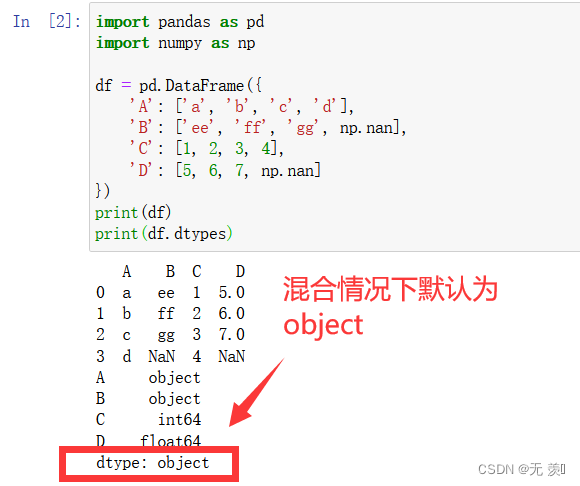（2）string类型需要通过设置`dtype`参数进行指定

``````# 方法1 ：dtype='string'
df = pd.DataFrame({

'A': ['a', 'b', 'c', 'd']}, dtype='string')
print(df.dtypes)

# 方法2 ： dtype=pd.StringDtype()
df = pd.DataFrame({

'A': ['a', 'b', 'c', 'd']}, dtype=pd.StringDtype())
print(df.dtypes)
``````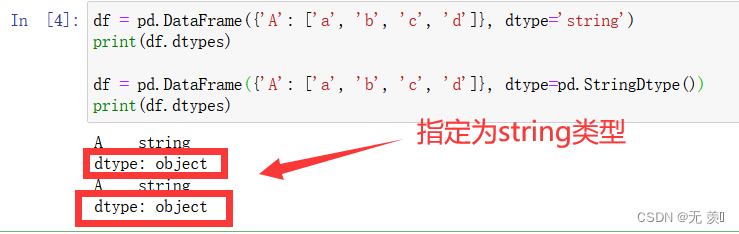## 2）类型转换

``````df = pd.Series({

'A': ['a', 'b', 'c', 'd']})
# 转换前
print(df)
print(df.dtypes)
# 转换后
df = df.astype("string")
print(df)
print(df.dtypes)
``````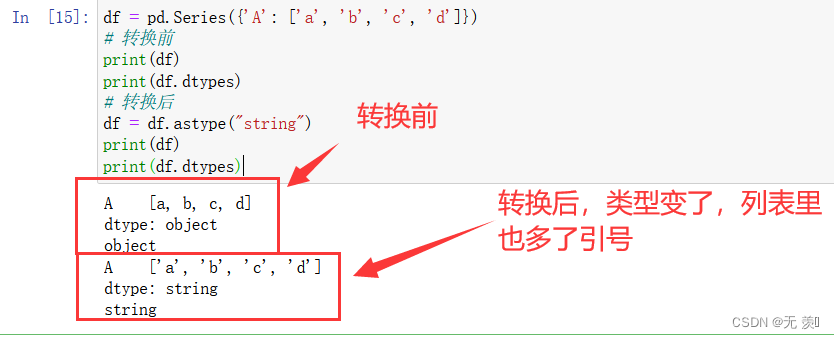``````import pandas as pd
import numpy as np

df = pd.DataFrame({

'A': ['a', 'b', 'c', 'd'],
'B': ['ee', 'ff', 'gg', np.nan],
'C': [1, 2, 3, 4],
'D': [5, 6, 7, np.nan]
})
print('类型转换前')
print(df.dtypes)

df = df.convert_dtypes()  # 智能数据类型选择
print('类型转换后')
print(df.dtypes)
``````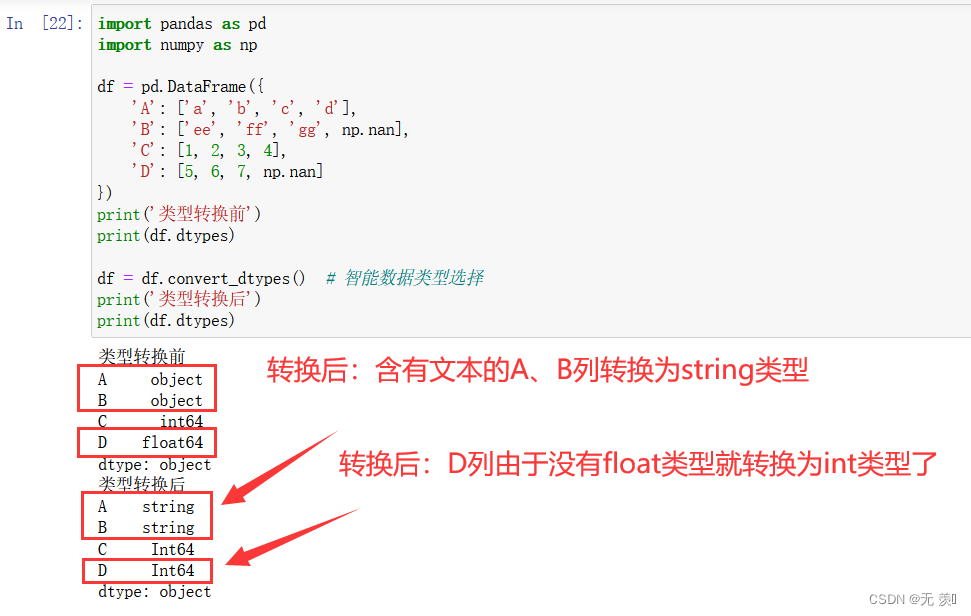## 3）类型区别

string类型和object类型区别如下：

• sting来说，返回数字输出的字符串访问器方法将始终返回可为空的整数类型；对于object来说，是 int 或 float，具体取决于 NA 值的存在
• 对于string类型来说，返回布尔输出的方法将返回一个可为空的boolean布尔数据类型；而object类型还是object

### 区别1：统计字符串时

• string类型的None返回NaN ，dtype为`Int64`；通过dropna()去除缺失值后dtype也是`Int64`
• object类型的None返回NaN，dtpye为`float64`；通过dropna()去除缺失值后dtype是`Int64`

string类型：

``````import pandas as pd
import numpy as np

s = pd.Series(['小明', '小红', None], dtype='string')
print("去除空值前：")
print(s)
print(s.str.count('小'))

print("去除空值后：")
s.dropna(inplace=True)
print(s)
print(s.str.count('小'))
``````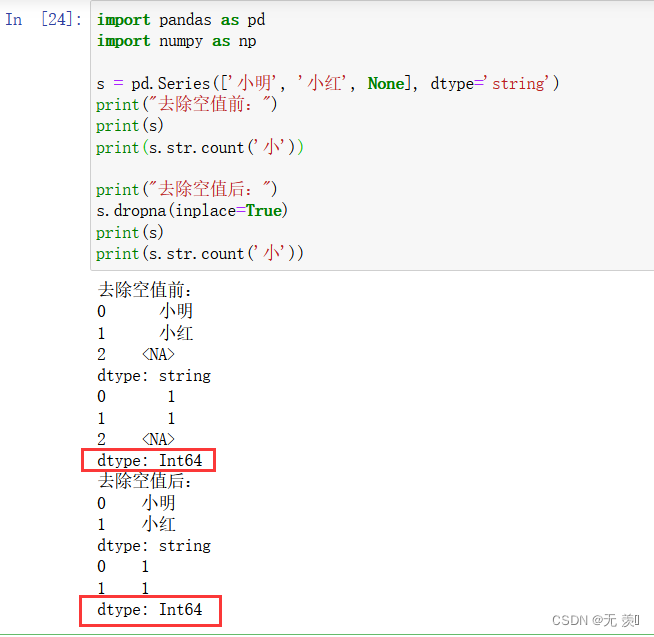object类型：

``````import pandas as pd
import numpy as np

s = pd.Series(['小明', '小红', None], dtype='object')
print("去除空值前：")
print(s)
print(s.str.count('小'))

print("去除空值后：")
s.dropna(inplace=True)
print(s)
print(s.str.count('小'))
``````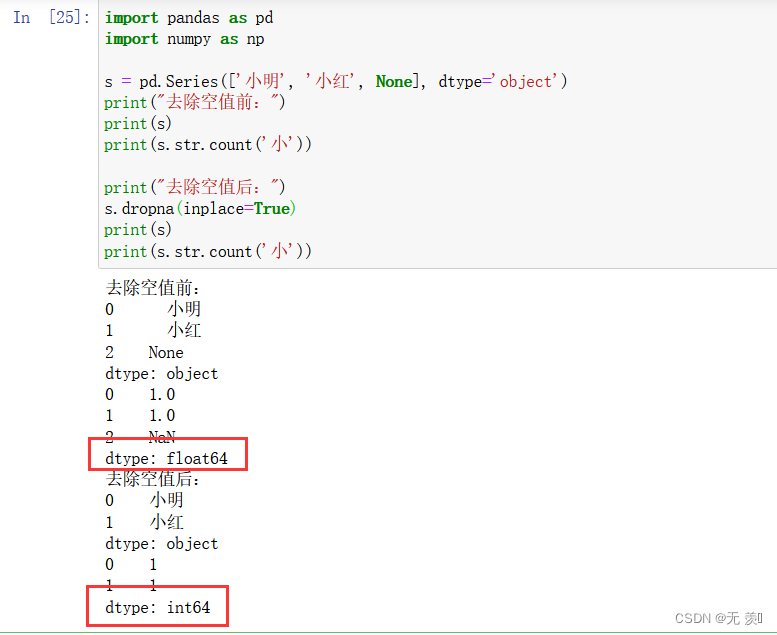### 区别2：检查字符串时

• string类型，则返回布尔类型，dtype= boolean，缺失值为NA
• object类型，虽然返回是布尔类型，但是dtype= object, None返回 None
``````import pandas as pd
import numpy as np

s = pd.Series(['小明', '小红', None], dtype='string')
print("string类型：")
print(s.str.isdigit())

s = pd.Series(['小明', '小红', None], dtype='object')
print("object：")
print(s.str.isdigit())
``````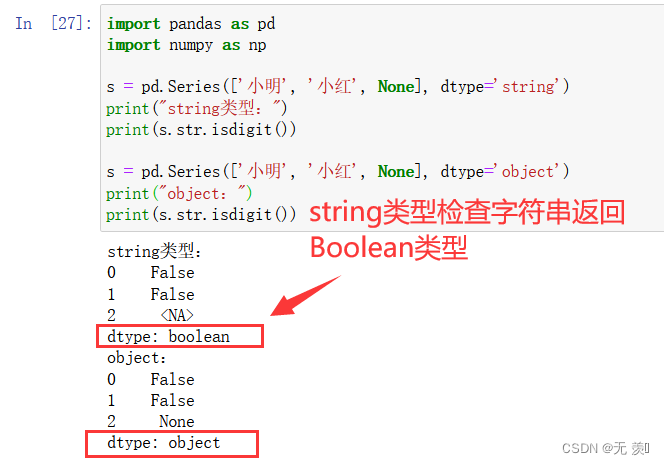# 2. Python字符串内置方法

## 1) 大小写转换

string.lower() 转换 string 中所有大写字符为小写
string.upper() 转换 string 中的小写字母为大写
string.capitalize() 把字符串的第一个字符大写
string.title() 把字符串的每个单词首字母大写
string.swapcase() 翻转 string 中的大小写

## 2) 文本对齐

string.ljust(width) 返回一个原字符串左对齐，并使用空格填充至长度 width 的新字符串
string.rjust(width) 返回一个原字符串右对齐，并使用空格填充至长度 width 的新字符串
string.center(width) 返回一个原字符串居中，并使用空格填充至长度 width 的新字符串

## 3）获取长度

len(string) 返回字符串的长度。

## 4）获取出现次数

count(string) 返回每个字符串元素出现的次数。

## 5）编码

encode(‘utf-8’) 字符编码，传递字符串

# 3. Pandas怎么使用内置方法？

• 在日常进行数据清洗、数据分析的过程中，经常需要对字符串类型数据进行处理。而pandas其内置的基于`Series.str`访问器的诸多针对字符串进行处理的方法，pandas特定的列经过str之后，就可以使用各种python常用的字符处理方法以及内置函数，可以帮助我们大大提升字符串型数据处理的效率。
• Pandas通过`.str`调用就可以在Series对象上使用字符串内置方法（pandas中的字符串处理函数以str开头），对数据框中的某一列进行操作，这种向量化的操作提高了处理效率。

## 1) 大小写转换

series_obj.str.lower() 转换 string 中所有大写字符为小写
series_obj.str.upper() 转换 string 中的小写字母为大写
series_obj.str.capitalize() 把字符串的第一个字符大写
series_obj.str.title() 把字符串的每个单词首字母大写
series_obj.str.swapcase() 翻转 string 中的大小写

``````import pandas as pd
import numpy as np

series_obj = pd.Series(['A', 'b', 'ABC', 'Abc', 'abc', 'This is abc', np.nan], dtype='string')
print(df)
``````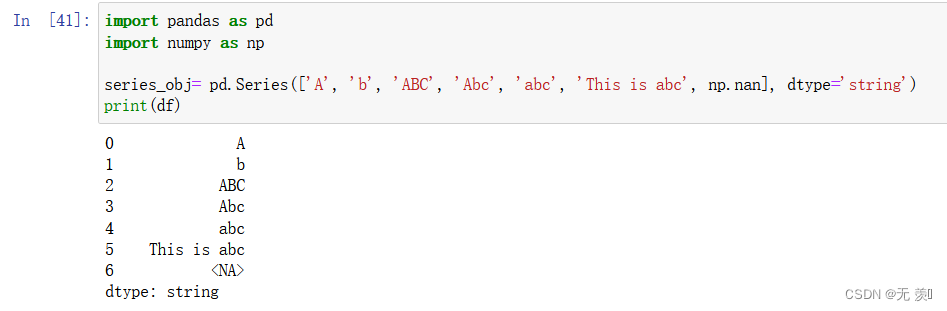1、转换 string 中所有大写字符为小写：

``````series_obj.str.lower()
``````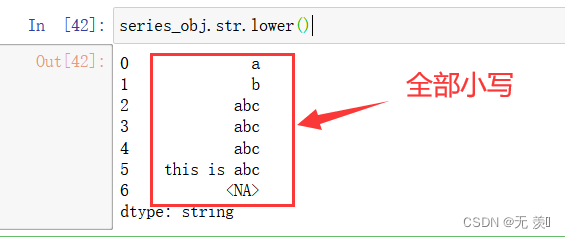2、转换 string 中的小写字母为大写：

``````series_obj.str.upper()
``````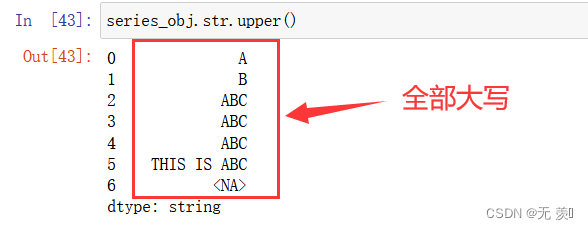3、把字符串的第一个字符大写：

``````series_obj.str.capitalize()
``````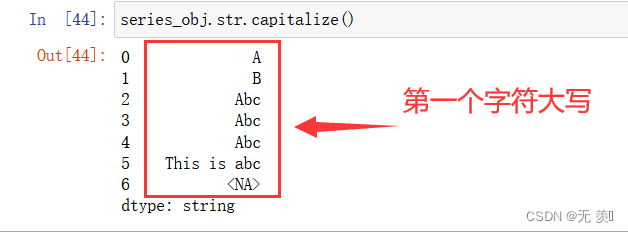4、把字符串的每个单词首字母大写（注意和capitalize的区别）：

``````series_obj.str.title()
``````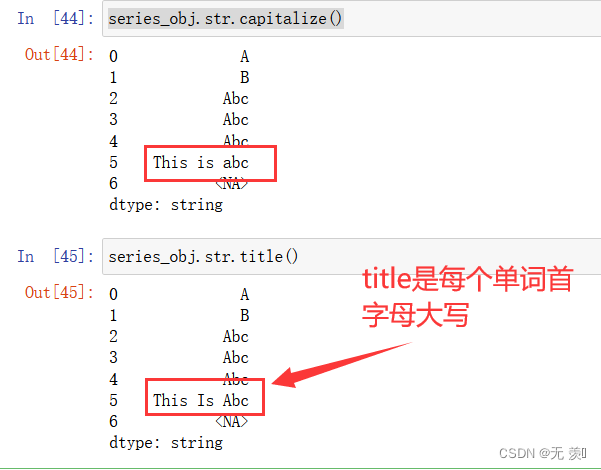5、翻转 string 中的大小写：

``````series_obj.str.swapcase()
``````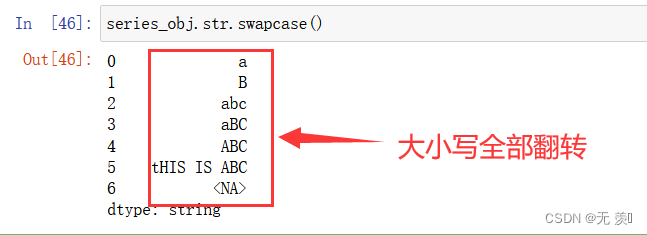## 2) 文本对齐

series_obj.str.ljust(width) 返回一个原字符串左对齐，并使用空格填充至长度 width 的新字符串
series_obj.str.rjust(width) 返回一个原字符串右对齐，并使用空格填充至长度 width 的新字符串
series_obj.str.center(width) 返回一个原字符串居中对齐，并使用空格填充至长度 width 的新字符串

1、 返回一个原字符串左对齐，并使用空格填充至长度 width 的新字符串：

``````# 左对齐：宽度为10，空余部分用 '-' 填充
series_obj.str.ljust(8, fillchar='-')
``````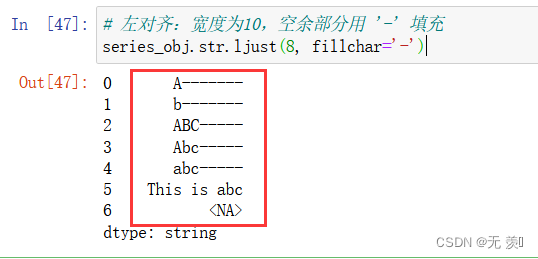2、返回一个原字符串右对齐，并使用空格填充至长度 width 的新字符串

``````# 右对齐：宽度为10，空余部分用 '-' 填充
series_obj.str.rjust(8, fillchar='-')
``````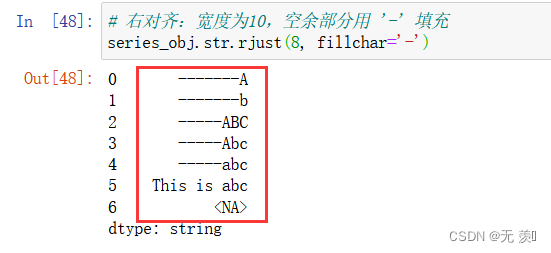3、返回一个原字符串居中对齐，并使用空格填充至长度 width 的新字符串：

``````# 居中对齐：宽度为10，空余部分用 '-' 填充
series_obj.str.center(8, fillchar='-')
``````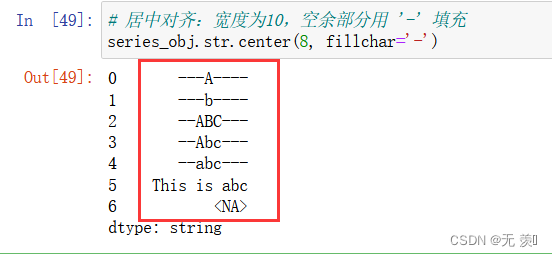## 3）获取长度

series_obj.str.len(string) 返回字符串的长度。
``````series_obj.str.len()
``````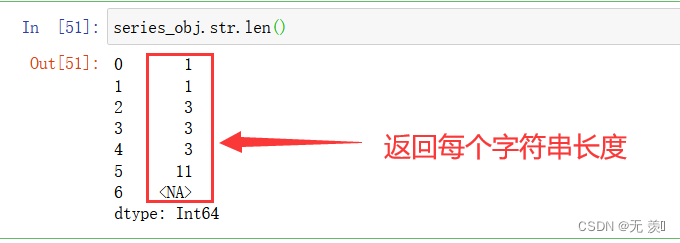## 4）获取出现次数

series_obj.str.count(string) 返回每个字符串元素出现的次数。

``````series_obj.str.count('A')
series_obj.str.count('a')
``````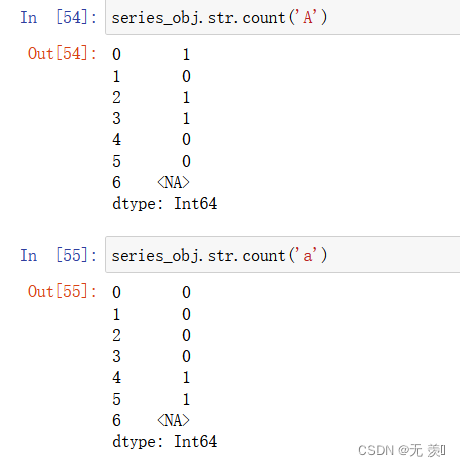## 5）编码

series_obj.str.encode(‘utf-8’) 字符编码，传递字符串

``````series_obj.str.encode('utf-8')
``````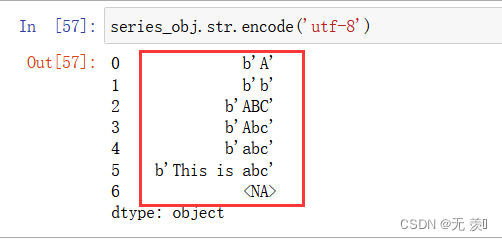# 4. 注意事项

1、`.str`访问器只能对Series数据结构使用。 除了常规列变量df.col以外，也可以对索引类型df.Index和df.columns使用

2、确保访问的对象类型是字符串str类型。 如果不是需要先astype(str)转换类型，否则会报错

3、某些方法不能在上stringSeries使用，如： `series_obj.str.decode()`，因为Series存储的是字符串而不是字节：

``````series_obj.str.decode('utf-8')
``````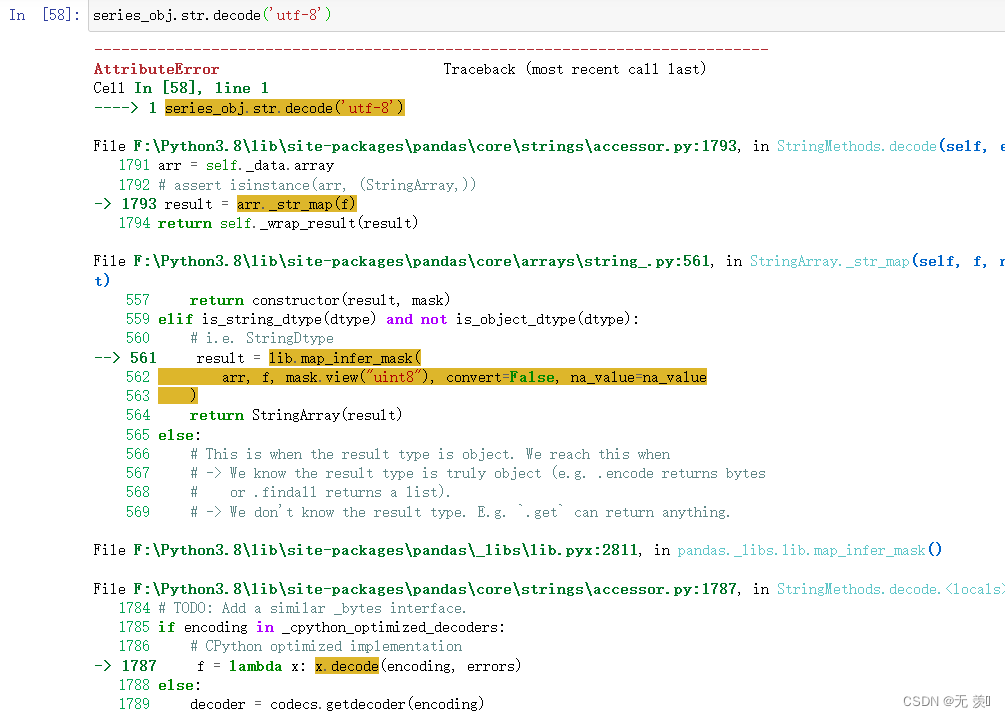4、访问器可以多个连接使用。 如`series_obj.str.lower().str.title()`，使用效果叠加：

``````# 先设置全部小写，然后设置首字母大写
series_obj.str.lower().str.title()
``````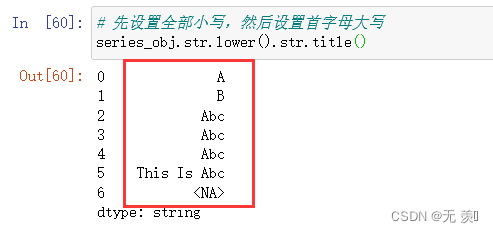# 代理IP

python离不开爬虫，最近有些想学爬虫的小伙伴问我，代理IP哪里找，博主自己用的是高匿稳定爬虫代理IP：神龙HTTP代理（需要的可自行点击了解）

# 书籍介绍

《PyTorch教程：21个项目玩转PyTorch实战》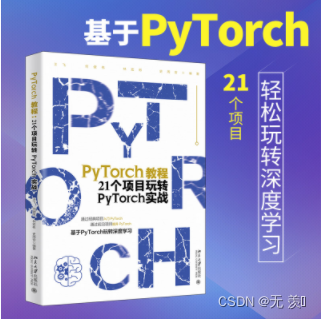PyTorch 是基于 Torch 库的开源机器学习库，它主要由 Meta（原 Facebook）的人工智能研究实验室开发，在自然语言处理和计算机视觉领域都具有广泛的应用。本书介绍了简单且经典的入门项目，方便快速上手，如 MNIST数字识别，读者在完成项目的过程中可以了解数据集、模型和训练等基础概念。本书还介绍了一些实用且经典的模型，如 R-CNN 模型，通过这个模型的学习，读者可以对目标检测任务有一个基本的认识，对于基本的网络结构原理有一定的了解。另外，本书对于当前比较热门的生成对抗网络和强化学习也有一定的介绍，方便读者拓宽视野，掌握前沿方向。